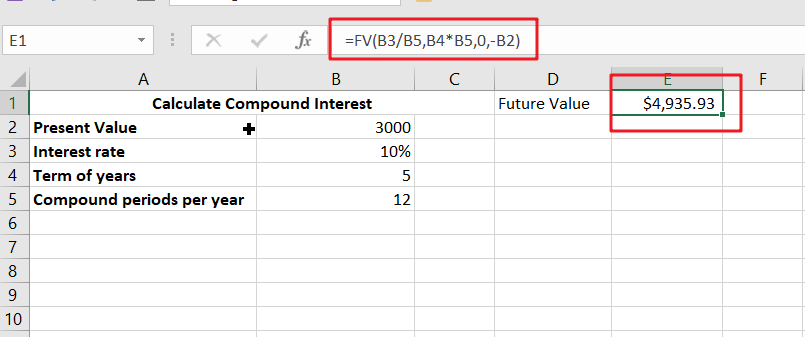# Calculate Compound Interest in Excel

This article will show you how to calculate compound interest in Excel. It will help you calculate the interest you will accrue on a given amount of money. There are several ways to calculate compound interest. One of the simplest is using the FV function. This function determines the future value of an investment. When calculating the future value of an investment, the FV function takes some arguments, the rate and the periodic payment, and the present value. The rate will determine how much interest is applied to payments made at the beginning of the period. If payments are made monthly, quarterly, or yearly, the FV function will return the future value of the investment.## What is Compound Interest?

Compound interest is a wonderful thing, especially if you’re looking to grow your savings. In this article we’ll explain what it means and how much better off our lives would be with some extra cash in the bank! So, create a new spreadsheet and enter the interest formulas you’d like to calculate to get started.

Compound interest is a financial tool that can make long-term investments more lucrative. In simple terms, it is interest earned on the principal plus interest. This cycle repeats itself to increase the value of the investment at maturity. Learning more about compounding can make your money work for you.

Compound interest is an important part of building wealth. Without the proper knowledge, it’s easy to get overwhelmed with debt. You could be drowning in debt in a few years if you don’t track compound interest. However, by learning how to calculate compound interest, you’ll be able to avoid costly mistakes and maximize your money. We’ll discuss how to calculate interest rates below.

## Generic Formula:

`=FV(rate,nper,pmt,pv)`

### Summary:

The FV function is used to calculate the future value of an investment based on the interest rate, the number of payments, and the period. The arguments used to compute the value of an investment are rate, period, nper, pmt, PV, and type. The FV function also allows you to change these inputs as required. For example, you can change the payments from beginning to end or at the end of a year. The formula in C10 is as follows:

`=FV(B3/B5,B4*B5,0,-B2)`### Syntax:

• Rate: Period rate
• NPER: Number of periods
• Pmt: Periodic payment
• PV: present value.

### Summing up:

In addition to calculating the interest on a loan, compound interest is an important tool in the financial world. It can help you manage your savings account and invest effectively. While compound interest can be a powerful force, it is also difficult for those who do not understand how it works. You can use the FV function for this purpose. You will be able to determine the future value of your savings based on the amount you initially put into them.

### Related Functions

• Excel FV function
The Excel FV function used to calculate the future value of an investment based on a constant interest rate. The syntax of the FV function is as below:=FV(rate,nper,pmt,[pv],[type])